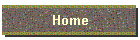Letter FrequenciesOne of the keys to solving a Rosettagram® is of course determining which language each version of the cipher is written in. Even without solving the cryptogram itself it is usually possible (given a large enough sample of the cipher) to figure out the language. One of the easiest things to do when sizing up a cipher is to do the math. That is 1. determine how many different symbols are used, actually list them and 2. count how many times each symbol is used in the ciphertext. Then 3. compare that set of values to the values of known letter frequency charts for known languages like German, English, Spanish, French, Latin, etc.

Listed below are letter frequency distributions for several languages. Experts on the subject point to   LANAKI  cryptography lessons as the place to start. The LANAKI lessons are online and can be accessed at the following website:  http://www.fortunecity.com/skyscraper/coding/379/lesson1.htm

Lessons 1, 5,6,&7 contain most if not all of the letter frequency charts you'll need, where else can you get a letter frequency chart  for Catalan?

English:

 Letter A B C D E F G H I J K L M N O P Q R S T U V W X Y Z % 8 1 3 4 13 3 1 6 7 4 2 7 8 3 7 6 9 3 1 2 2
```ENGLISH:   13  9  8   7   6    4  3     2     1    <1
E  T  AO  INR HS   DL CFPU  MWY   BGV  JKQXZ```
```            10  9    7    6    4   3      <2
LATIN:      I   E   UTA  SRN  OM  CPL    (bal)```
```            18   8    7    6   5  4   3   2    <1
FRENCH:     E    AN  RSIT  UO  L  D  CMP  VB   F-Y```
```            18  11  8  7    5     4    3    2     <1
GERMAN:     E   N   I  RS  ADTU  GHO  LBM  CW    (bal)```
```            13  12  11  9  7    6   5    3     2   <1
ITALIAN:    E   A   I   O  L   NRT  SC  DMO'U  VG   (bal)```
```            20  10   7   6  5   4   3      2       <1
DUTCH:      E   N   IAT  O  DL  S  GKH  UVWBJMPZ   (bal)```
```            13   9  8   7   5    4   3    1    <1
SPANISH:    EA   O  S  RNI  DL  CTU  MP   GYB  (bal)```

Now how to use these numbers, LANAKI explains what they mean below:

The following is an excerpt from LANAKI Lesson 1

Friedman was the first to employ the principle that English
Letters are mathematically distributed in a unilateral
frequency distribution:

13 9 8 8 7 7 7 6 6 4 4 3 3 3 3 2 2 2 1 1 1 - - - - -
E T A O N I R S H L D C U P F M W Y B G V K Q X J Z

That is, in each 100 letters of text, E has a frequency (or number of appearances) of about 13; T, a frequency of about 9; K Q X J Z appear so seldom, that their frequency is a low decimal.

Other important data on English ( based on Hitt's Military Text):

6 Vowels: A E I O U Y                                                  =  40 %

20 Consonants:
5 High Frequency (D N R S T)                                  =  35 %
10 Medium Frequency (B C F G H L M P V W)       =  24 %
5 Low Frequency (J K Q X Z)                                    =   1 %
====
100.%